﻿ 锰基载氧体生物质化学链气化反应热力学研究 Thermodynamics Research of Chemical Looping Gasification of Biomass Based on Mn-Based Oxygen Carrier

Hans Journal of Chemical Engineering and Technology
Vol.08 No.03(2018), Article ID:24804,7 pages
10.12677/HJCET.2018.83018

Thermodynamics Research of Chemical Looping Gasification of Biomass Based on Mn-Based Oxygen Carrier

Hongjie Wang, Kun Wang*, Jia Wei, Kemu Zhang, Yunhong Su

School of Metallurgy, Northeastern University, Shenyang Liaoning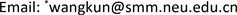Received: Apr. 19th, 2018; accepted: May 1st, 2018; published: May 9th, 2018ABSTRACT

Based on the principle, this paper adopts gasification and air reactors to produce the syngas with CO and H2 as the main compositions. Using HSC simulation software, the effects of reaction temperature and pressure, ratio of H2O to oxygen carrier, ratio of oxygen carrier and biomass on the composition and concentration of the syngas were simulated. The results show that the volume fraction of CO and H2 increases with the increasing of gasification reaction temperature. The CO and H2 in the syngas decrease gradually with the increasing of gasification reaction pressure. As the molar ratio of H2O to oxygen carrier increases, the content of CO + H2 decreases significantly. As the molar radio of Mn2O3 to biomass increases, the content of CO increases significantly, the content of H2 decreases gradually, and the contents of CH4 and CO2 increase slightly. The parameters are determined as follows: gasification temperature is 1000˚C; gasification pressure is 1 atm; the molar radio of H2O to oxygen carrier is 0.5; the molar radio of oxygen carrier to biomass is 0.10.

Keywords:Biomass, Chemical Looping Gasification, Thermodynamics, Reaction Conditions1. 引言

2. 生物质化学链气化原理

${\text{C}}_{\text{n}}{\text{H}}_{\text{2m}}{\text{O}}_{\text{x}}=\text{char}+\text{tar}+\text{syngas}$ (1)

$\text{CO}\left(\text{g}\right)+{\text{3Mn}}_{\text{2}}{\text{O}}_{\text{3}}={\text{CO}}_{\text{2}}\left(\text{g}\right)+{\text{2Mn}}_{\text{3}}{\text{O}}_{\text{4}}$ (2)

$\text{CO}\left(\text{g}\right)+{\text{Mn}}_{\text{2}}{\text{O}}_{\text{3}}={\text{CO}}_{\text{2}}\left(\text{g}\right)+\text{2MnO}$ (3)

${\text{H}}_{\text{2}}\left(\text{g}\right)+{\text{3Mn}}_{\text{2}}{\text{O}}_{\text{3}}={\text{H}}_{\text{2}}\text{O}+{\text{2Mn}}_{\text{3}}{\text{O}}_{\text{4}}$ (4)

${\text{H}}_{\text{2}}\left(\text{g}\right)+{\text{Mn}}_{\text{2}}{\text{O}}_{\text{3}}={\text{H}}_{\text{2}}\text{O}+\text{2MnO}$ (5)

${\text{CH}}_{\text{4}}\left(\text{g}\right)+{\text{3Mn}}_{\text{2}}{\text{O}}_{\text{3}}={\text{2H}}_{\text{2}}\left(\text{g}\right)+\text{CO}\left(\text{g}\right)+{\text{2Mn}}_{\text{3}}{\text{O}}_{\text{4}}$ (6)

${\text{CH}}_{\text{4}}\left(\text{g}\right)+{\text{4Mn}}_{\text{2}}{\text{O}}_{\text{3}}={\text{2H}}_{\text{2}}\text{O}+{\text{CO}}_{\text{2}}\left(\text{g}\right)+\text{8Mn}$ (7)

$\text{C}+{\text{3Mn}}_{\text{2}}{\text{O}}_{\text{3}}=\text{CO}\left(\text{g}\right)+{\text{2Mn}}_{\text{3}}{\text{O}}_{\text{4}}$ (8)

$\text{C}+{\text{2Mn}}_{\text{2}}{\text{O}}_{\text{3}}={\text{CO}}_{\text{2}}\left(\text{g}\right)+\text{4MnO}$ (9)

$\text{CO}\left(\text{g}\right)+{\text{H}}_{\text{2}}\text{O}\left(\text{g}\right)={\text{H}}_{\text{2}}\left(\text{g}\right)+{\text{CO}}_{\text{2}}\left(\text{g}\right)$ (10)

$\text{C}+{\text{H}}_{\text{2}}\text{O}\left(\text{g}\right)={\text{H}}_{\text{2}}\left(\text{g}\right)+\text{CO}\left(\text{g}\right)$ (11)

$\text{C}+{\text{CO}}_{\text{2}}\left(\text{g}\right)=\text{2CO}\left(\text{g}\right)$ (12)

${\text{CH}}_{\text{4}}\left(\text{g}\right)+{\text{H}}_{\text{2}}\text{O}={\text{3H}}_{\text{2}}\left(\text{g}\right)+\text{CO}\left(\text{g}\right)$ (13)

3. 模拟部分

4. 模拟结果分析

4.1. 气化温度对合成气占比的影响Figure 1. Principle of chemical looping gasification of biomassTable 1. The industry and elemental analysis of biomass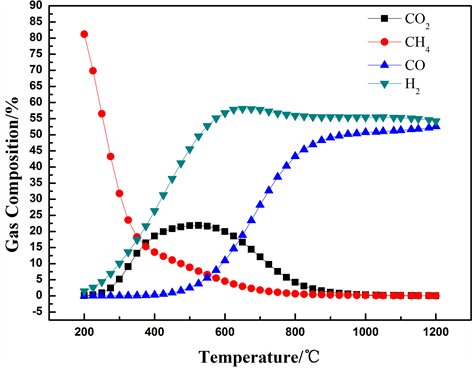Figure 2. The yield of syngas varies with temperature

4.2. 气化压强对合成气生产的影响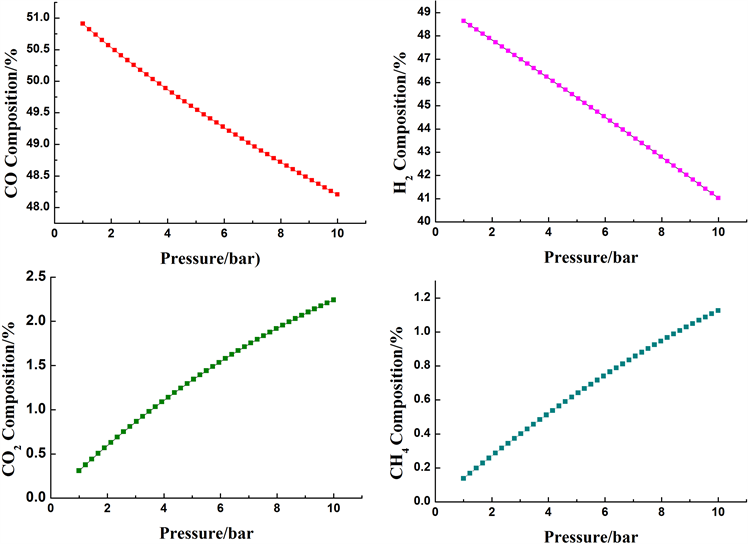Figure 3. The yield of syngas varies with pressure

4.3. 水蒸气/载氧体对合成气占比的影响

4.4. 载氧体/生物质对合成气占比的影响

Mn2O3作为必要的热和氧的载体，载氧体/生物质必然影响生物质气化合成气中各占比。图5给出了载氧体/生物质对合成气各占比的影响。由图分析表明，H2随载氧体/生物质增加而逐渐下降，相反地，CO占比不断升高，CO2和CH4占比稍有升高。这是由于较多的载氧体与合成气体发生氧化还原(2, 3, 4, 5, 6, 7)，产生较多的CO，并消耗大量的CH4。考虑到CO的增加和吉布斯自由能最小化促进了生物质气化(11, 12, 13)向逆反应进行，促进水汽变换反应(10)向正反应进行，导致CH4占比略有增加。考虑到H2热值高并且无污染，在较低的载氧体添加比例下进行气化会生成更多H2。载氧体/生物质选择为0.10。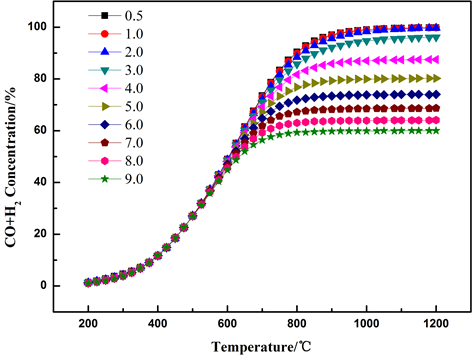Figure 4. Influence of molar ratio of H2O and oxygen carrier on concentration of CO + H2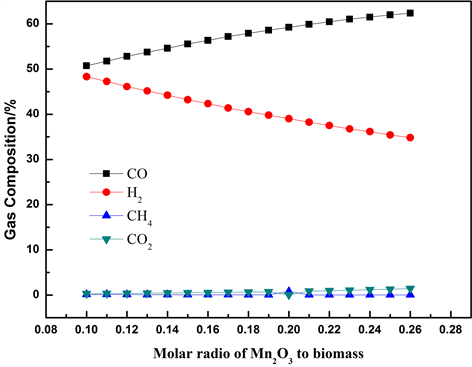Figure 5. Influence of the molar radio of Mn2O3 to biomass on concentration of outlet gas

5. 结论

1) 随着温度升高，合成气中CH4不断减少，CO2先增后降，而CO、H2则不断增加，故选择1000℃为气化温度。

2) 随着压强增大，合成气中CO、H2略有减少；CO2和CH4稍有增加，故选择在常压下进行。

3) 随着水蒸气/载氧体比例增大，气化反应器出口处CO + H2连续下降。确定水蒸气/载氧体为0.5。

4) 随着载氧体/生物质比例增大，H2不断减少，CO不断增大，CO2和CH4则稍有升高。确定载氧体/生物质为0.10。

Thermodynamics Research of Chemical Looping Gasification of Biomass Based on Mn-Based Oxygen Carrier[J]. 化学工程与技术, 2018, 08(03): 151-157. https://doi.org/10.12677/HJCET.2018.83018

1. 1. 董玉平, 景元琢,郭飞强. 生物质热解气化技术[J]. 中国工程学, 2011, 13(2): 44-49.

2. 2. 李季, 孙佳伟, 郭利, 等. 生物质气化新技术研究进展[J]. 热力发电, 2016(45): 1-6.

3. 3. 曹俊, 钟文琪, 金保昇, 等. 流化床生物质气化过程的三维数值模拟[J]. 工程热物理学报, 2014(6): 1114-1118.

4. 4. 张瑞华. 我国农村推广秸秆类生物质气化集中供气技术探讨[J]. 环境保护科学, 2005(2): 67-69.

5. 5. Ryden, M., Lyngfellt, A. and Mattisson, T. (2008) Chemical-Looping Combustion and Chemical-Looping Reforming in a Circulating Fluidized-Bed Reactor Using Ni-Based Oxygen Carriers. Energy and Fuels, 22, 2585-2597.

6. 6. Sheng, L.H., Gao, Y. and Xiao, J. (2007) Simulation of Hydrogen Production from Biomass Gasification in Interconnected Fluidized Beds. Biomass and Bioenergy, 32, 120-127.

7. 7. 秦晓楠. 铝基Pd与Ni催化剂生物质气催化燃烧的实验研究[D]: [硕士学位论文]. 武汉: 华中科技大学, 2009.

8. 8. 吴创之, 阴秀丽, 罗曾凡, 等. 生物质富氧气化特性的研究[J]. 太阳能学报, 1997(3): 2-7.

9. 9. 诸林, 邓亚欣, 陈虎, 等. 基于化学链制氧的生物质气化产氢工艺[J]. 过程工程学报, 2017, 17(2): 306-312.

10. 10. 黄振, 何方, 李海滨, 等. 天然铁矿石为氧载体的生物质化学链气化制合成气实验研究[J]. 燃料化学学报, 2012, 40(3): 300-308.

NOTES

*通讯作者